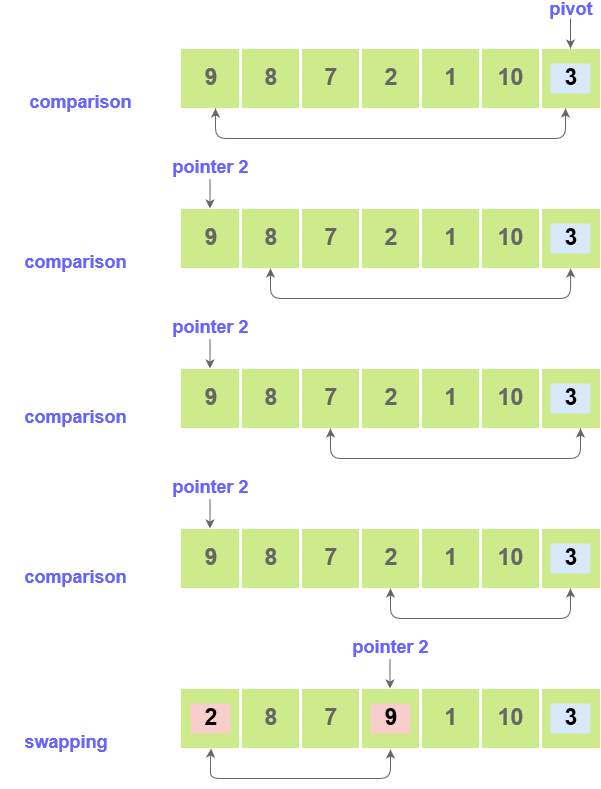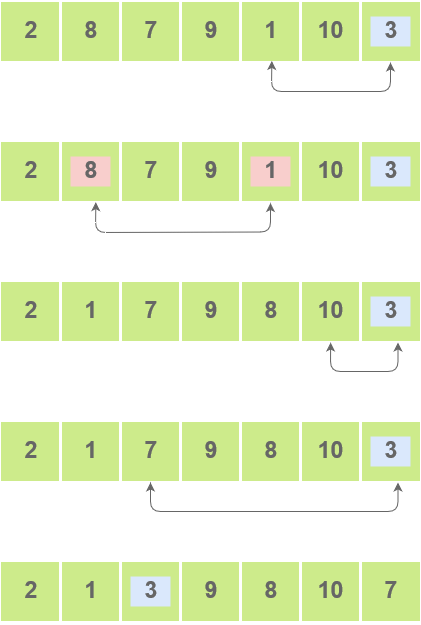# Quick Sort Partitioning Algorithm

## Quick Sort Partitioning Algorithm

Quick sort is one of the fastest and most widely used algorithm to sort an array. It is based on Divide & Conquer technique and works in a similar way to merge sort by recursively sorting the left part and right part of arrays.

The central idea of quick sort algorithm is to choose a pivot element and then “partitioning the array” based on this pivot. After partitioning the array, the elements which are smaller than pivot will go to the left of the pivot and the elements which are greater than pivot will go to the right of the pivot.

After we are done with the partition, we can sort the left part and the right part of the array recursively as shown in the pseudo code below:

### Quick Sort Pseudo Code

``````// Sorts the part of array b/w index start and end
QuickSort(A, start, end)
{
// Choosing last element as pivot
pivot = A[end]
// Partitions the array A based on the pivot and returns the position of the pivot
p = partition(A, start, end, pivot)

// Now recursively sort the left part
QuickSort(A, start, p -1 );
QuickSort(A, p + 1, end);
}``````

To implement the partition function used above, we can use Two Pointer Method.

We make make two indexes `i` and `j`. `i` will always point towards the boundary of the elements which are less than the `pivot`. Since, we don’t know where does the boundary of the pivot end we will initialize `i = -1`

After that we will start iterating over the array and keep on swapping elements at `A[i]` with current element, if the `j`th element is less than pivot.

The pseudocode for the above method is as given below:

### Quick Sort Partitioning Pseudo Code

``````    i = -1
pivot = A[end]
for j = 0 to n - 1 {
if (A[j] < pivot) {
i++
Swap(A[i], A[j])
}
}
Swap(A[i+1], A[end])``````

### Quick Sort Partitioning VisualizationScroll to Top

### Full Stack Integrated Bootcamp Free Trial

• Please enter a number from 7000000000 to 9999999999.